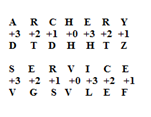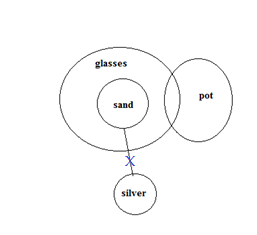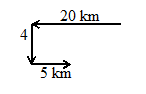# SSC CGL 2018 Practice Test Papers | Reasoning Ability (Day-20)

Dear Aspirants, Here we have given the Important SSC CGL Exam 2018 Practice Test Papers. Candidates those who are preparing for SSC CGL 2018 can practice these questions to get more confidence to Crack SSC CGL 2018 Examination.

[WpProQuiz 2955]

Click “Start Quiz” to attend these Questions and view Explanation

1) If ARCHERY is coded as DTDHHTZ then how the word SERVICE will be coded?

a) UGSVLEF

b) VGSVLEF

c) UGSYREW

d) OPSVQWE

2) which of the following condition follows the Statement

Some glasses are pots. No silver is Sand. All Sand are glasses.

a) Some silver are pot.

b) Some pot is sand.

c) Both a and b

d) Neither a nor b

3) Raj walks 20 km straight and then turns left and walks for 4 km and again left and walks for 5 km. If he is facing east direction then toward which direction he started his journey?

a) East

b) West

c) North

d) South

4) DF: 52::HI: ?

a) 145

b) 200

c) 235

d) 155

5) Find the wrong response in given series.

45, 21, 73, 22, 46, 31

a) 45

b) 46

c) 21

d) 73

6) aaa_bcbb_c _d_ _cd_e

a) aabbcd

b) bbccdd

c) bbbcce

d) bbcccd

7) Find the missing number in the series.

12, 14, __, 97, 393

a) 41

b) 43

c) 31

d) 33

8) Find the missing number in the series.

 45 47 51 62 67 92 ? 36 72

a) 45

b) 30

c) 22

d) 33

9) A word is represented by only one set of numbers as given in any one of the alternatives. The set of numbers given in any one of the alternatives. The sets of numbers given in the alternatives are represented by two classes of alphabets as in two matrices given below. The columns and rows of matrix I are numbered from 0 to 4 And that matrix II are numbered from 5 to 9. A letter from these matrices can be represented first by its row and next by its column. Eg: A can be represented by 21. Similarly you have to identify the set for the word ‘YARD’

Matrix I

 0 1 2 3 4 0 D Z T P U 1 S Q M R Y 2 B A C S V 3 T W D G F 4 O L H U A

Matrix II

 5 6 7 8 9 5 U E Y X R 6 D U O U K 7 Y A K L U 8 R D T Y J 9 V N M I D

a) 75, 44, 58, 66

b) 88, 76, 13, 99

c) 95, 68, 42, 14

d) 75, 76, 13, 44

10) Find the odd one out.

a) Goa

b) Pune

c) Bhuvaneshwar

d) DispurDF=4^2+6^2=52

HI=8^2+9^2=145

45, 21=>4×5+1=21

73, 22=>7×3+1=22

Instead of 46, 56 or 65 should be there

Series is aaabbcbbbccdcccdde

12×1+2=14

14×2+3=31

31×3+4=97

97×4+5=393

47-45 = 2, 47+2^2 = 51

67-62 = 5, 67+5^2 = 92

36-30 = 6, 36+6^2 = 72